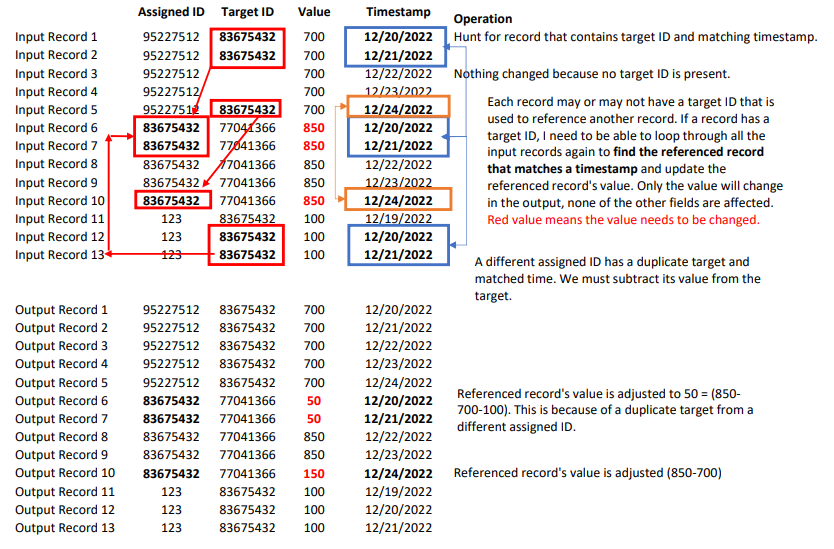Customer Portal

# Access Multiple Records and Update Values Dynamically?

•Lukas Cholasta
• Official comment

HI Justin,

I know we have sorted this out via a direct support ticket but I'm just adding the solution here for completeness sake.

Attached is the example graph, let my try to explain what it does. So I have prepared a sample input file based on your description. This is read and then the first Map component creates two artificial joining keys (joinedKeyAT and joinedKeyTT), these allows for simpler calculations and joining later on. Then this enriched data is copied into two sort components that just sort these two flows on each of the new fields. The Denormalizer component takes the TT flow and creates groups based on the joinedKeyTT field so that we can calculate the final values (per each group that matches the (assignedID = targetID and Timestamp = Timestamp) that need to be subtracted from the other flow which represents the assigned ID group. Then the ExtMergeJoin component simply subtracts these values. The second Map component simply cleans up the artificial keys that we have previously created.

Kind regards,

Lukas

•Hi,

As each record contains its own ID and ID of the referenced record, you can copy (with the SimpleCopy component) the data flow onto another edge and use a joiner component (e.g., ExtHashJoin component) to join these records based on those two IDs. After joining, the joiner components produce two output flows. The first output port is used for the joined records, while the second is for the unjoined ones. Therefore, you need to gather these two flows using the SimpleGather component.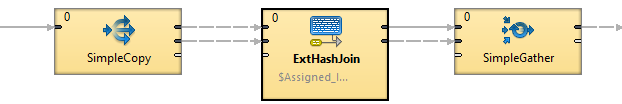Set the join key as follows (The Assigned_ID describes the ID of the current record while the Target_ID describes which record's value is to subtract):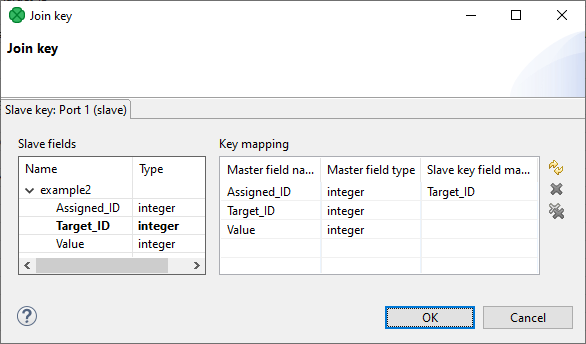For the subtraction part, you can subtract the values in the Transform of the ExtMergeJoin component.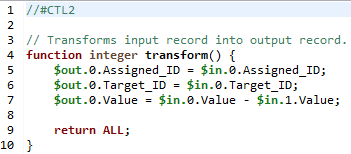Best,

•Justin Tran

I just wanted to follow up with a quick question: is there a way to perform the subtraction in this component for duplicate slave values as well? What I mean by this is that if there are multiple records with different Assigned_IDs that point to the same Target_ID, is there a way in this component to subtract the value from the Target_ID more than just once?

Thank you for any insight you can give, and please let me know if there's anything I can clarify.

Cheers,

Justin Tran

•Hi Justin,

I am afraid that doing all that in the ExtHashJoin component would not be possible. This sounds more like a use case for the Denormalizer component usage. In theory, if you have your Target_ID and Assigned_IDs already joined (via ExtHashJoin) you will be able to perform the deduction inside the Denormalizer's CTL code. Then, you would output a single record for each TargetID with the calculated value (and possibly a list of denormalized Assigned_IDs).

As an example, let's say that your data looks like this: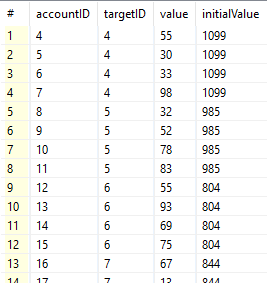where "initialValue" represents the initial value of the given targetID and "value" represents the value of an accountID that should be deducted from the initial value.

In that case, your Denormalizer key would be targetID and the CTL code could look something like this:

`//#CTL2integer[] allAccIDs;integer calculatedValue;function integer append() {    append(allAccIDs, \$in.0.accountID);    calculatedValue = calculatedValue + \$in.0.value;    return OK;}function integer transform() {    \$out.0.accountID = allAccIDs;    \$out.0.targetID = \$in.0.targetID;    \$out.0.value = \$in.0.initialValue - calculatedValue;    return OK;} function void clean() {     allAccIDs = [];     calculatedValue = 0; }`

The resulting data would look like this: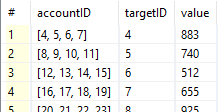If this is not applicable to your use case I'd suggest either of the two options below:

• Provide short and comprehensive sample input data and sample of the desired output data (basically what you are trying to achieve.
• Open a support ticket via the Customer Portal and provide a snippet of the actual input data and again, a sample of the desired output data.
•Justin Tran